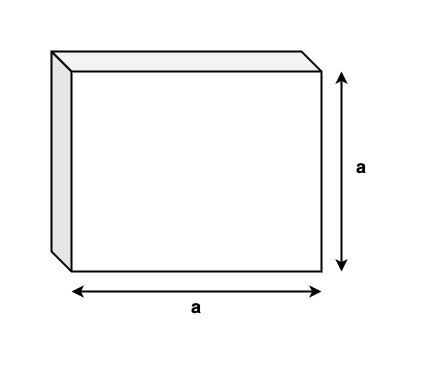# Swift Program to calculate the area of Cube

This tutorial will discuss how to write a Swift program to find the area of the cube.

A cube is solid three dimensional shape with six square faces and all the sides of the cube are of equal length(that means Length = Breadth = Width). It is also known as regular hexahedron.The area of a cube is known as the total space enclosed inside the boundaries of the cube. The area of cube is of two types-

• Total surface area
• Lateral surface area

## Total Surface Area

The total area occupied by all the six faces of the cube is known as the total surface area of the cube.

### Formula

Following is the formula of the total surface area of the cube −

Area = 6(side)2


Below is a demonstration of the same.

Input

Suppose our given input is −

Side = 5


Output

The desired output would be −

Area = 6 * (5)2 = 150.
Area = 150


### Algorithm

Following is the algorithm.

• Step 1 − Declare a variable with user-defined/pre-defined value. This variable stores the side of the cube.

• Step 2 − Declare another variable named “cubeArea” to store the surface area of the cube.

var cubeArea = 6 * (cubeSide * cubeSide)

• Step 3 − Print the output.

### Example

The following program shows how to find the total surface area of the cube.

import Foundation
import Glibc

var cubeSide : Int = 7

// Finding the total surface area of cube
var cubeArea = 6 * (cubeSide * cubeSide)

print("Side - ", cubeSide)
print("So, Area of the cube- ", cubeArea)


### Output

Side -  7
So, Area of the cube-  294


Here in the above code, we calculate the total surface area of the cube using the following formula −

var cubeArea = 6 * (cubeSide * cubeSide)


And display the output that is 294.

## Lateral Surface area

The total area occupied by the side or lateral faces of a cube is known as the lateral surface area.

### Formula

Following is the formula of the lateral surface area of the cube −

Area = 4(side)2


Below is a demonstration of the same −

Input

Suppose our given input is −

Side = 3


Output

The desired output would be −

Area = 4 * (3)2 = 36.
Area = 36


### Algorithm

Following is the algorithm −

• Step 1 − Declare a variable with user-defined/pre-defined value. This variable stores the side of the cube.

• Step 2 − Declare another variable named “cubeArea” to store the lateral area of the cube.

var cubeArea = 4 * (pow(cubeSide, 2))

• Step 3 − Print the output.

### Example

The following program shows how to find the lateral surface area of the cube.

import Foundation
import Glibc

var cubeSide : Double = 8.0

// Finding the lateral surface area of cube
var cubeArea = 4 * (pow(cubeSide, 2))

print("Side - ", cubeSide)
print("So, Area of the cube- ", cubeArea)


### Output

Side - 8.0 So,
Area of the cube- 256.0


Here in the above code, we calculate the lateral surface area of the cube using the following formula −

var cubeArea = 4 * (pow(cubeSide, 2))


And display the output that is 256. Here we use pow() function to calculate the power of the side.

Updated on: 25-Aug-2022

182 Views